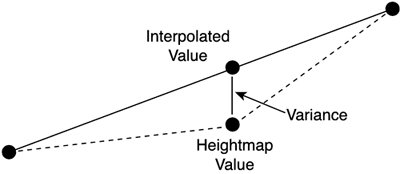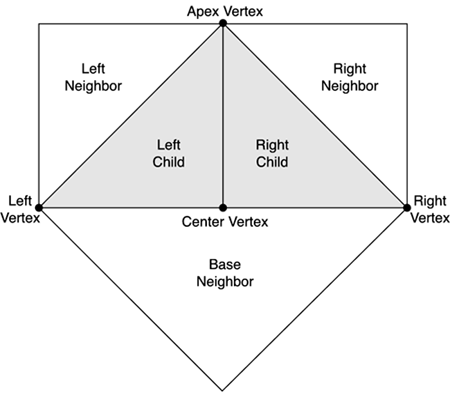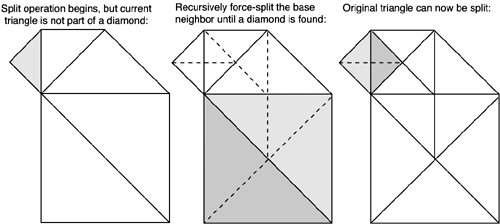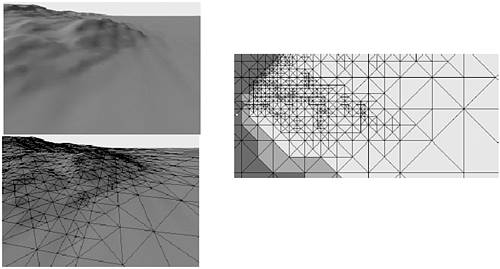# ROAM

ROAM is one of the most powerful outdoors approaches devised to date. The algorithm was designed by a team working at the Lawrence Livermore National Laboratory, led by Mark Duchaineau. It became popular in games such as Tread Marks by Seumas McNally and has been used in many others since then. The algorithm combines a powerful representation of the terrain with a dynamic LOD rendering approach that changes the resolution of the terrain as we move around. What follows is a thorough description of the ROAM algorithm. However, I must provide a word of warning. ROAM, like BSPs, is a very complex algorithm. I suggest you take the following sections one step at a time, making a drawing along the way to ensure you understand it. For more information, I recommend that you review the original paper, which provides many implementation details, and the Gamasutra article by Bryan Turner. Both are referenced in Appendix E, "Further Reading." Having these papers at hand will prove very useful if you are serious about ROAM.

ROAM is a two-pass algorithm that allows very fast terrain rendering. ROAM does not have a geometric representation of the terrain beforehand: It builds the mesh along the way, using precomputed measures of detail to know where it should refine further. On the first pass, terrain data is scanned into a bintree, and a view-independent error metric is computed. This metric will allow us to detect zones in which more detail is needed. Then a second pass constructs a second bintree, which performs the actual mesh construction and rendering.

#### Pass One: Construct the Variance Tree

In the first phase, we will build a representation of the detail in the terrain. To do so, we need to establish a metric for detail. Several metrics are proposed in the original paper, and others were devised later. One of the most popular error metrics is called the variance, which was introduced in the game Tread Marks, and is defined inductively. For a leaf node (a bintree that is one pixel across), we define the variance as the difference in height between the interpolated hypotenuse and the real value of the heightmap for that point. Thus, we compute the hypotenuse midpoint and compare that to the real value of the terrain (see Figure 14.6).

##### Figure 14.6. Variance in ROAM for a leaf node.For any node in the tree that is not a leaf, the variance is the maximal variance of any descendant from that node. Thus, we need to explore the terrain fully until we reach the base nodes, each representing just one pixel from our heightmap. We compute basic variances at these nodes, and then propagate them to build nested variances in the backtracking phase. The variance of the root node will be the maximal variance of any of the triangles that are derived from it. Here is the algorithm to compute the variance:

` int CalcVariance(tri) { int RealHeight = the map height at the middle of the hypotenuse int AvgHeight = the average of the real heights at the two ends of the hypot int v = abs( RealHeight - AvgHeight ) if tri->LeftChild is valid    {    v = max( v, CalcVariance(tri->LeftChild) )    } if tri->RightChild is valid    {    v = max( v, CalcVariance(tri->RightChild) )    } return v } `

As you can see, we need a data structure for the nested variances. The logical structure would be a binary tree, so we can reproduce the nested behavior of variances. This tree is often mapped to a static array for faster access in the rendering phase because we will need to access this structure frequently.

Thus, the first phase of any ROAM implementation basically builds the nested variance data structure, which provides us with useful information for the mesh reconstruction and rendering phase.

#### Pass Two: Mesh Reconstruction

Once the variance tree is built, we can take advantage of it to build the mesh for rendering. To do so, we build a BTT of geometry, and then expand it more or less using the variance hints.

The BTT is just a regular tree that is used to store triangles. The root node represents a large triangular area of the terrain, and each descendant covers a subset of that initial zone. Because it is a triangular shaped tree, some magic will be needed to support quadrilateral zones (usually two trees joined at the root). Each node of the triangle tree approximates a zone of the terrain by storing only its corner values. If the vertices inside the zone are not coplanar to these corners, we can use the variance tree to determine how much detail still exists inside that subzone, and thus make a decision to supersample it further. You can see this construct along with a complete BTT in Figure 14.7.

##### Figure 14.7. BTT, shown from the top down.The key idea of the BTT construction is to use the view-independent error metric, coupled with a view-dependent component to decide how deep we must propagate into the tree while building the mesh in the process. As with geomipmaps, we set a maximum threshold and recurse until a node generates an error smaller than the threshold. But we know beforehand that this process will indeed introduce gaps and cracks because neighboring triangles need not be of the same resolution level. Thus, sometimes their edges simply will not match. Geomipmapping solves this issue by welding patches of different resolution together. ROAM works in a different direction. Whenever we encounter a discontinuity, we oversample neighboring patches to make sure they are sown together properly. To do so, we need to label each side of a triangle and use some rules that guarantee the continuity of the mesh.

We will call the hypotenuse of the triangle the base and call the other two sides the left and right sides, depending on the orientation of the triangle. The same terminology applies to neighbors. Thus, we can talk about the base neighbor (which is along the hypotenuse) and so on (see Figure 14.8). If you analyze several bintrees, you will discover that neighbors from a triangle can only exist in specific configurations. For example, the base neighbor will either be from the same level as the original node or from the next coarser level. Left and right nodes, on the other hand, can either be located at the same level or one level finer at most.

##### Figure 14.8. Triangles and their labels.From this fact, we can derive three rules of splitting that govern when and how we can recurse further into the tree. The first rule applies to those nodes that are part of a diamond (thus, connected to a base neighbor of the same level). In these cases, we must split the node and the base neighbor to avoid creating cracks. Both nodes will split in sync, and continuity will be preserved. A second case happens when a node is at the edge of the mesh. This is the trivial case because we can keep splitting even if we cause side effects. The third case deals with nodes that are not part of a diamond. In this case, we must first-force split the base neighbor before we split our current node, and thus preserve continuity. Here is a summary of these rules:

• If part of a diamond, split the node and the base neighbor.

• If at the edge of a mesh, split as needed.

• If not part of a diamond, force-split the base neighbor before splitting the current node.

So fundamentally, the run-time algorithm is as follows: Traverse the bintree and couple the view-independent variance with a view-dependent component (usually, the distance to the viewer) so a view-dependent metric of error is returned. We then want to recurse until the error level for that node is lower than a fixed threshold. We must follow the three splitting rules as explained in the previous paragraph to ensure we create a continuous mesh. For an example of the splitting at work, take a look at Figure 14.9.

##### Figure 14.9. Left: Split begins; we are not part of a diamond, so if we split the shaded triangle, continuity will be lost. Center: We recursively split (using the rules) the base neighbor so we gain the vertex, which we will use to (right) split the current node.Notice that the mesh will be quite conservative. Because of rules 1 and 3, we will sometimes be forced to split a node further than required, so its resolution will be higher. This is because the node might have a highly detailed neighbor, and because we need to recurse the neighbor further, we also end up recursing the node more than is needed. Notice, however, that the opposite is not true: The mesh will never be coarser than the fixed threshold.

Nodes pending a split operation are usually arranged in a priority queue, called the split queue. This is sorted by priority, which is generally the view-dependent error. Then, we start at the base triangulation of the bintree (the root, which covers the whole terrain with a single triangle) and trigger the following greedy algorithm:

` T = base triangulation while T is too inaccurate       identify highest priority node from T (node with biggest error)       force-split using the rules       update queue:             remove the node that has just been split             add the new nodes resulting from the split end while `

So we store nodes where the algorithm is standing currently. We compute the incurred error of the triangulation as the biggest error in the nodes, and if the overall error is too large, we select the node with the highest priority (biggest error) and force split it using the splitting rules previously explained. We then remove the offending node, substitute it with those nodes arising from the split, and start over. If we implement the splitting rules correctly, this generates an optimal, continuous mesh with error levels below the fixed bound.

This is the simplest type of ROAM and is usually referred to as split-only ROAM. It computes a continuous mesh for a terrain heightfield, but it comes with a host of problems that must be addressed to reach reasonable performance. ROAM is a hard algorithm because its base implementation is not fast enough for more or less anything.

#### Optimizations

As you have seen, ROAM is a CPU-intensive algorithm. In fact, its performance is seldom limited by the sheer number of triangles to render, but by the binary triangle tree construction and traversal. Experience shows that, in most cases, a split-only ROAM will not be fast enough to render large, detailed pieces of terrain. We will now cover some optimization techniques that will multiply the performance of any split-only ROAM implementation.

The first change to the ROAM engine is to divide the terrain into sectors so the bintree does not cover the whole map. Rather, we would have a 2D matrix of bintrees, each covering parts of the terrain. This makes trees less deep and also simplifies tessellation. ROAM is still too expensive to be computed per frame, and besides, doing so is a complete waste of resources.

Imagine that the player is standing still facing the landscape. Do we really need to recompute the tessellation per frame? Obviously not, and that's why ROAM is usually implemented on a frame-coherent basis. The terrain is only recomputed every few frames, usually using an interleaved bintree policy as well. We do not recompute each bintree each frame, but rather cycle through the different trees frame by frame (or even every number of frames). So if we have an NxM array of bintrees, we compute a bintree's tessellation once every NxM frames. This greatly reduces the CPU hit of the tessellation. A similar approach is to make ROAM retessellation dependent on the player's change of position and orientation. We can force a recalc if and only if the player has moved more than X meters from the last recalc position or has rotated more than Y degrees. If coupled with the sector-by-sector approach outlined earlier, this should definitely put an end to most of our performance concerns. Take a look at a ROAM algorithm in action in Figure 14.10.

##### Figure 14.10. ROAM at work. Left: Camera view of the level with the mesh (above) and the wire frame views (below). Right: The same frame, seen from a top-down camera. Notice how the blending of the view-dependent and the view-independent components preserves detail.Another completely different line of thought is to work on frustum culling. Remember that we are working with a BTT, so there's some interesting issues that arise from the frustum cull phase. The most popular technique is to label each node in the bintree with six flags, one for each half space delimiting the frustum. This effectively means storing a Boolean for each one of the six clipping planes to determine if the triangle is completely inside or if it's either partially or totally outside. Then, a global IN, OUT, and DON'T-KNOW (for triangles part-in, part-out) label is assigned to the node. Because the tree is hierarchical, we can update this information easily on a frame-to-frame basis. A node IN means all subnodes until we reach the leaves that are all IN, for example, and the opposite is also true for OUT nodes. Because we recalc the culling information, only some portions of the tree will have changed, and we will devote our efforts to those. Suddenly, a node that was IN or OUT (and required no further analysis) will turn to DON'T-KNOW because it will be partly inside, partly outside. We then need to scan only that subtree to keep the information current. Then, for painting purposes, all we need to do is render those nodes that are IN or DON'T-KNOW and prune OUT nodes directly.

Another improvement to the core ROAM algorithm is to stripify triangles as we retessellate. This is obviously not for the faint of heart, because real-time stripification is a complex problem. In the original paper, a suboptimal incremental tristripper tries to create strips as nodes are split. Results show splits between four and five vertices on average, which also helps reduce the amount of data sent over the bus.Core Techniques and Algorithms in Game Programming2003
ISBN: N/A
EAN: N/A
Year: 2004
Pages: 261